Father and son

Father is 48 years old, son 23. When will be the father twice older than a son?

Result

x =  2

Solution:

48+x = 2*(23+x)

x = 2

x = 2

Calculated by our simple equation calculator.

Leave us a comment of this math problem and its solution (i.e. if it is still somewhat unclear...):Be the first to comment!To solve this verbal math problem are needed these knowledge from mathematics:

Do you have a linear equation or system of equations and looking for its solution? Or do you have quadratic equation?

Next similar math problems:

1. Six yearsIn six years Jan will be twice as old as he was six years ago. How old is he?
2. The ship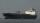The ship went upstream speed of 20 km/h in relation to water. River flows at speed 10 km/h. After half an hour, he stopped and returned downstream to the starting point. How long it took way back when even if downstream speed of ship is 20 km/h in relation
3. Two looms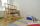On two different looms with different performance can be make required quantity of textile in 6 hours when both work together. On the first machine this quantity of the textile can make in 10 hours. How long should take make same quantity of textile on a s
4. 12 Moons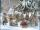Good Marry came to ask twelve moons for help with collecting strawberries. All twelve moons 1200 strawberries picked in 20 minutes. But lazy months July and August cease tear after 5 minutes. How many minutes in total will take collect strawberries?
5. Fifth of the numberThe fifth of the number is by 24 less than that number. What is the number?
6. Unknown numberIdentify unknown number which 1/5 is 40 greater than one tenth of that number.Number 118 divide into two addends, so first addend is 69 greater than 75% of the second addend.
8. CandyPeter had a sachet of candy. He wanted to share with his friends. If he gave them 30 candies, he would have 62 candies. If he gave them 40 candies, he would miss 8 candies. How many friends did Peter have?
9. Simple equationSolve for x: 3(x + 2) = x - 18
10. EquationSolve the equation: 1/2-2/8 = 1/10; Write the result as a decimal number.
11. Workers 2Worker dug the trench for 7 hours, another worker for 10 hours. They recruited to work even one worker more. Digging should be done for 2 hours. For how long would dig third worker alone?
12. Welders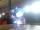Welder do the job for six hours, the second one for 8 hours. How long do the job third welder if all together can do job in 2 hours?
13. Painters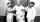One painter could paint a school for 7 days. The second one for 6 days. It was necessary to paint the school in two days. Next painter was invited and all worked together. How long would this painter painted the school itself?
14. Collection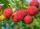John has collected half a bucket of raspberries in 45 minutes, Eva collects the whole bucket in an hour. How many minutes it would take to fill a bucket if the two children work together?
15. Two tributaries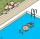Two tributaries of the pool fill it in 10 hours. One of the tributaries would fill 15 hours. How long would fill the first tributary?
16. MeatHalf a kilogram of pork and three-quarters of a kilogram of beef cost total 5.1 USD. One quarter of a kilogram of beef and 1 kilogram of pork cost 4.9 USD. Determine the price of 1 kg of each type of meat.
17. Sprayers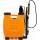The vineyard can be sprayed first sprayer in 5 hours and with the second in 8 hours. How long would it take if we use sprayers both at once?